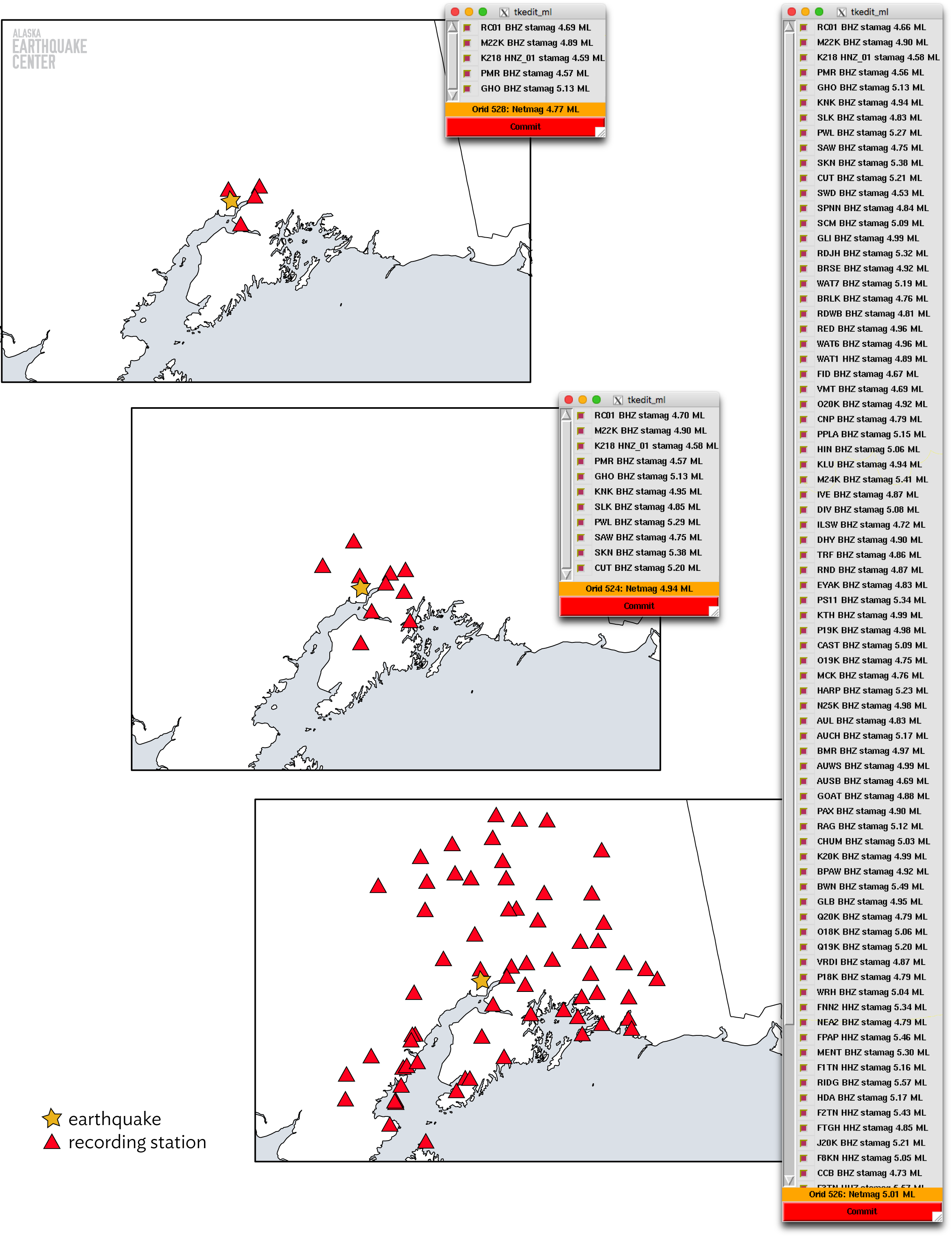Why magnitudes evolve in the minutes after an earthquake

If you've ever read one of our old information releases, you might have noticed the phrase "The magnitude and location may change slightly as additional data are received and processed." The words probably make sense, but the reasons are a bit subtle. Ultimately, there are usually two reasons for a magnitude value changing: either we reported a different magnitude type, or an analyst reviewed the earthquake, updating the location and/or the stations used in the magnitude calculation.

### Using a different magnitude type

In the early days of modern seismology, there was only a single way of computing magnitude - the Richter magnitude. This method still works quite well today (renamed to local magnitude, Ml), but only for magnitudes of less than about 6. At earthquakes of this size, the scale will systematically underestimate the true magnitude, and the effect just gets worse as the magnitude grows.

Since then, many other types of magnitudes have been devised (see https://earthquake.usgs.gov/learn/topics/mag-intensity/magnitude-types.php). They all attempt to replicate the number produced by the Richter calculation, but use different combinations of signal frequencies or seismic phases. Of all the types, the moment magnitude, Mw, is generally considered to be the best way of computing magnitudes that we currently have. This is because it represents the true amount of energy released by an earthquake, instead of approximating the energy by looking at various waveform amplitudes.

At the Earthquake Center, we compute local magnitudes for all earthquakes, regardless of size. Most of the time, this is the magnitude that you will see reported. However, if the earthquake is of sufficient size (usually 5.5, but always if it is 6.5 or greater), then we instead use the Mw solution as soon as it is available.

### Updating location or stations

While switching magnitude types sometimes happens, most magnitude changes are the result of an update to the earthquake location and changing the stations that we used in the magnitude calculation (for a quick overview of the earthquake location procedure, see https://earthquake.alaska.edu/three-stages-earthquake-locations-automatic-reviewed-revised and watch for our upcoming story with more information on how we compute automatic locations).

As noted in the section above, the primary magnitude that the Earthquake Center calculates is a local magnitude. Calculating this type of magnitude is a very simplistic procedure, relying only on the amplitude of shaking recorded on a station and the station's distance from the earthquake source. Given these two pieces of information, a magnitude value is calculated at that single station. This process is repeated for every station used to locate the earthquake. In the end, we are left with a list of (hopefully) multiple magnitudes. A seismologist then takes the average magnitude value (weighted based on distance to the earthquake source, closer stations weighing more heavily into the average) across all the stations, removing any notable outliers, and returns the final magnitude value. In the figure here, you can see examples of the differences in an earthquake's magnitude based on the stations recording it. The lists show the magnitude for each station and the weighted average. The magnitude increases from 4.77, calculated for 5 stations up to 5.01 calculated for 90 stations.

Since the magnitude calculation is highly dependent on the station's distance from the source, changing the earthquake's hypocenter can have a large impact on the final magnitude. Notice how station RC01 in the figure has a slightly different value in all three calculations. The largest changes usually happen when our automated system locates an earthquake very poorly. For example, say the automated system wrongly puts a small earthquake deep at 100 kilometers, when it is in fact only 5 kilometers deep. Since the distance to each station is based on three dimensions, a large depth would correspond to every station being farther away from the source in the magnitude calculation. Every station would then report a magnitude that is higher than in reality. When a seismologist notices the mislocation and corrects the location to its correct value of 5 km, that distance decreases and the magnitude would become much lower.

### What happens when a magnitude changes?

Most of the time, not much. Our systems watch for updated magnitudes and automatically update the information on our website and the USGS website, along with any related products such as ShakeMaps, with the new magnitude. Most of the time, the value change is small or the earthquake is small and was not felt, so the changes go unnoticed by nearly everyone. If you do happen to notice a change, though, hopefully now you have a better idea as to why it happened.These three panels illustrate how the magnitude calculated changes based on the stations used. Analysts have the ability to toggle off stations with notably different magnitudes from the average.